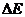Chapter 10, Problem 78AP### Introductory Chemistry: A Foundati...

9th Edition
Steven S. Zumdahl + 1 other
ISBN: 9781337399425

#### Solutions

Chapter
Section### Introductory Chemistry: A Foundati...

9th Edition
Steven S. Zumdahl + 1 other
ISBN: 9781337399425
Textbook Problem
18 views

# A system releases 213 kJ of heat and has a calculatedof −45 kJ. How much work was done on the system?

Interpretation Introduction

Interpretation:

The work done on the system should be calculated.

Concept Introduction:

The internal energy, ΔE of a system is sum of potential energy and kinetic energy of all the particles. This can also be written in terms of work and heat as follows:

ΔE=q+w

Here, ΔE is change in internal energy, q is heat and w is work.

Explanation

Given Information:

The energy releases is 213 kJ and the value of change in internal energy is -45 kJ.

Calculation:

The internal energy is related to heat and work done as follows:

ΔE=q+w

Since, the heat is released thus, the value is -213 kJ

On rearranging and putting the values,

w=ΔE

### Still sussing out bartleby?

Check out a sample textbook solution.

See a sample solution

#### The Solution to Your Study Problems

Bartleby provides explanations to thousands of textbook problems written by our experts, many with advanced degrees!

Get Started